Next: Q-learning in Generalized -MDPs Up: MDPs in Varying Environments Previous: Generalized -MDPs

## Asymptotic Boundedness of Value Iteration

In this section we prove a generalized form of the convergence theorem of Szepesvári and Littman's (for the original theorem, see Appendix A). We do not require probability one uniform convergence of the approximating operators, but only a sufficiently close approximation. Therefore the theorem can be applied to prove results about algorithms in generalized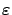-MDPs. Our definition of closeness both for value functions and dynamic-programming operators is given below.

Let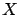be an arbitrary state space and denote bythe set of value functions. Let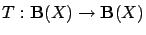be an arbitrary contraction mapping with unique fixed point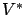, and let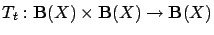be a sequence of random operators.

Definition 3.1   A series of value functions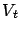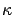-approximates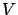with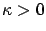, if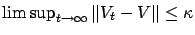with probability one.

Definition 3.2   We say that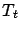-approximates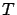atover, if for any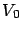and for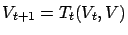,-approximates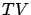overwith probability one.

Note thatmay depend on the approximated value function, unlike the previous example in Equation 4.-approximation of value functions is, indeed, weaker (more general) than probability one uniform convergence: the latter means that for all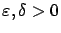there exists a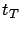such that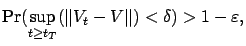whereas an equivalent form of-approximation is that for all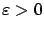there exists asuch that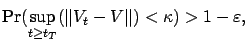andis fixed.

Theorem 3.3   Letbe an arbitrary mapping with fixed point, and let-approximateatover. Letbe an arbitrary value function, and define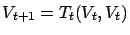. If there exist functions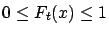and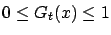satisfying the conditions below with probability one
1. for all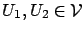and all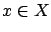,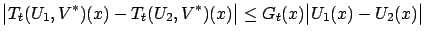2. for all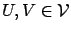and all,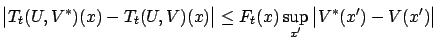3. for all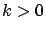,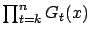converges to zero uniformly inas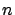increases;2 and,
4. there exists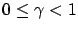such that for alland sufficiently large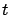,then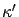-approximatesover, where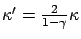.

Proof. The proof is similar to that of the original theorem. First we define the sequence of auxiliary functions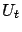by the recursion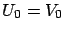,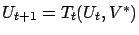. Since-approximatesat,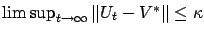, i.e., for sufficiently large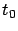and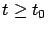,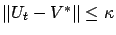. Let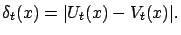Forwe have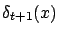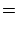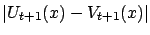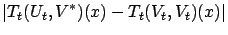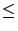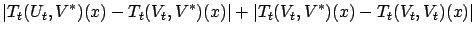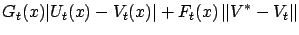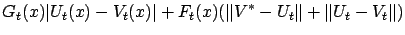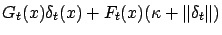Then, by Lemma C.1 (found in the appendix), we get that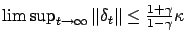. From this,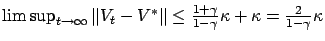.Next: Q-learning in Generalized -MDPs Up: MDPs in Varying Environments Previous: Generalized -MDPs